MIKE'S SIMPLIFIED TRANSFORMER THEORY

The following information is based on building transformers using E-I laminations.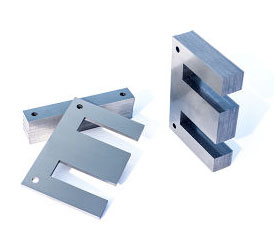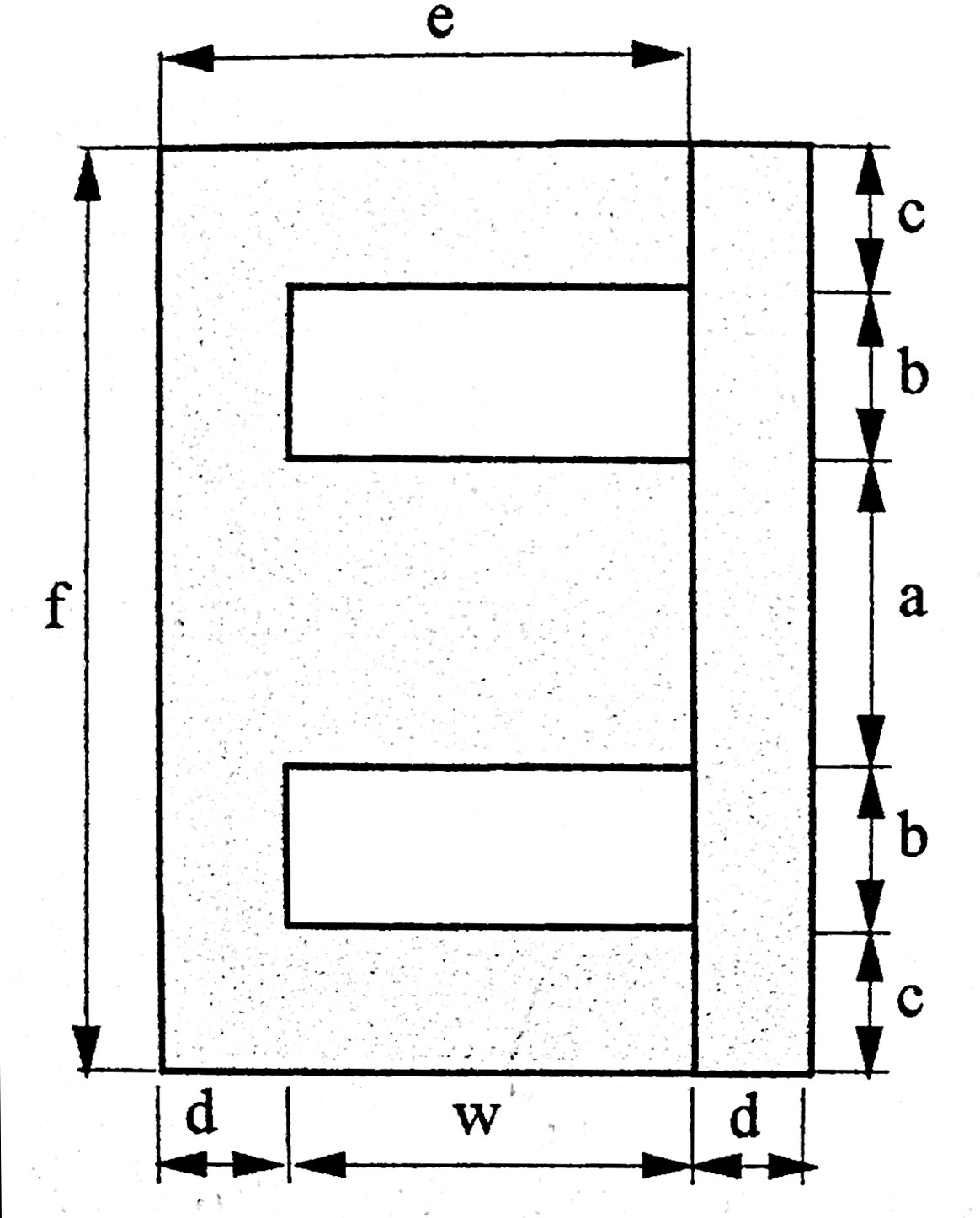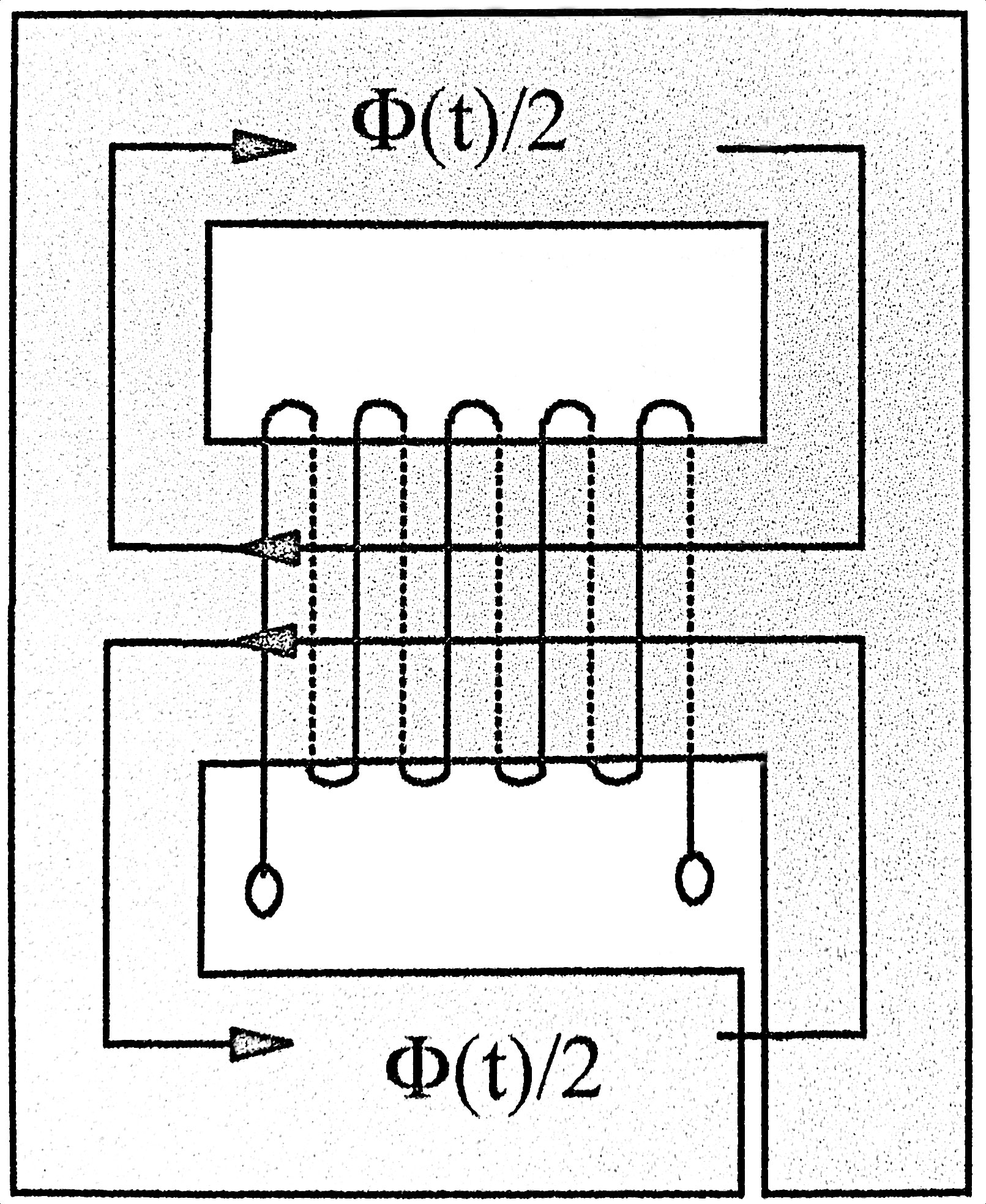The photo on the left are shows E and I pieces individually and stacked to form a core. The center diagram shows the different dimensions used on the E lamination for the table below. To the right. The right diagram shows the flow of flux through the center leg and dividing to flow in the outer legs and then back to the center leg. This is the mean magnetic length discussed below.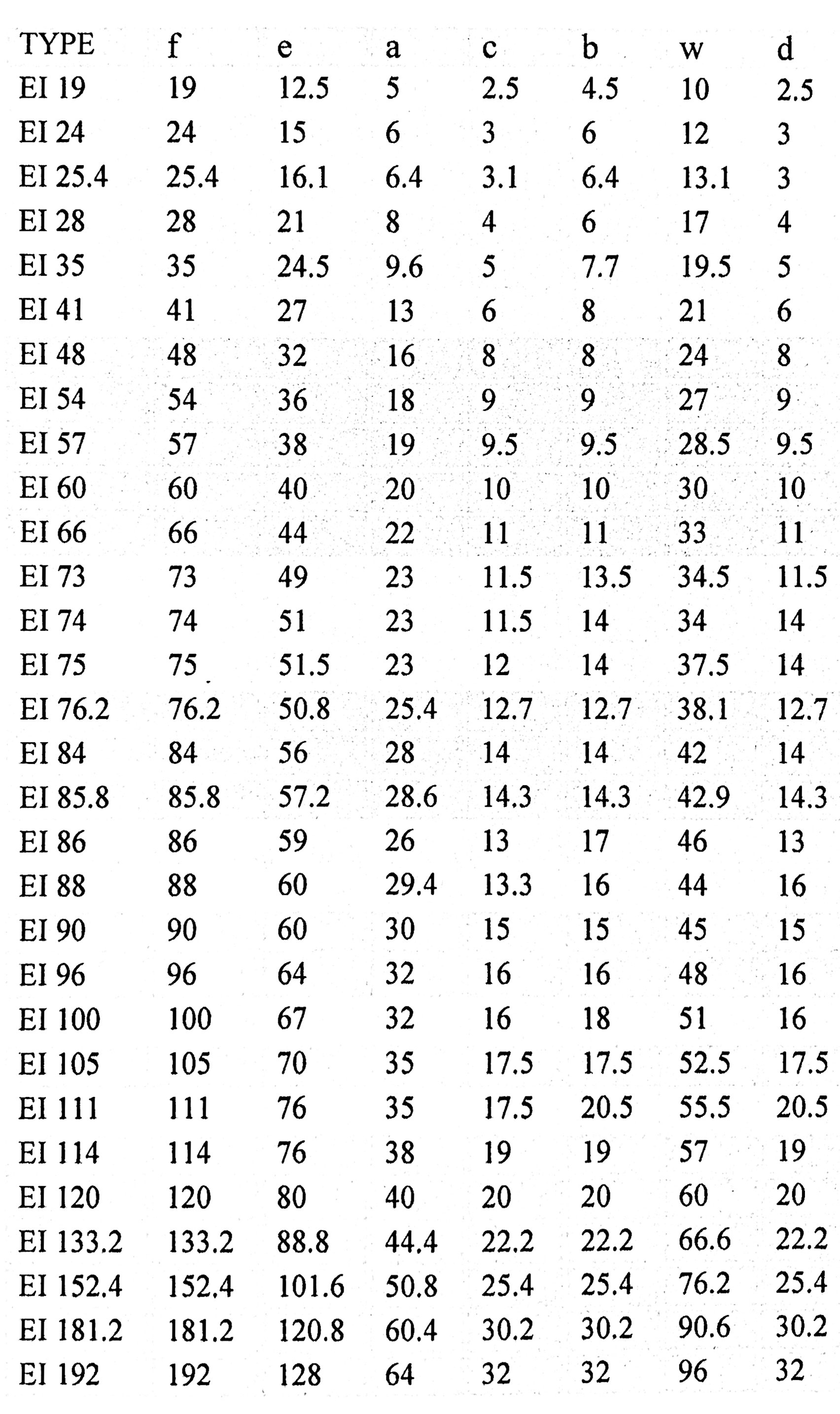The standard chart for E-I laminations is shown here. It is applied to the center diagram above.

The mean magnetic length ℓMP discussed below is calculated from the table using the following formula:

MP = 2(b+e-d) + πc

Example: for an EI66 lamination:
MP = 2(11+44-11) + 11π = 122.5mm = 12.25cm.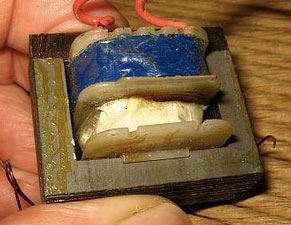This is a nearly finished E-I transformer. A bobbin with wire wound around it is fit onto the center leg of the E-piece, then the stack of I pieces (darker brown in photo to the left) is put in place.

The Magnetizing Force or Magnetic Field Strength H is defined as:
(1)   H(t) = i(t) * N / ℓMP     where N=number of turns, ℓMP = mean magnetic length, described below

The flux density B is the Magnetizing force H multiplied by the permeability μ of the core, where μ = μRO
μR = relative permeability = 1 for air, much higher than 1 for ferromagnetic materials.
μ0 = absolute permeability = 4π*10-7, so
(2)   B(t) = μROH(t)

Magnetic flux Φ is the flux density B x the cross sectional area A of the core.
(3)   Φ(t) = B(t) *A
(4)   Φ(t) = μROH(t) * A
(5)   Φ(t) = μRO*i(t)*N*A/ℓMP

Now the induced voltage is proportional to the derivative of the flux Φ(t) and is negated.
V(t) = -N * dΦ(t)/dt
Using equation (5):
V(t) = -N2RO/ℓMP * di/dt
Now, this is the familiar inductance equation with:
(6)   L = N2RO/ℓMP

For any sinusoidal wave,
(7)   Φ(t) = Φmaxsinwt

(8)  V(t) = -NΦmaxw*cos(wt)       by differentiating (7)

As in equation (7), V(t) can be expressed as V(t) = Vmax*sin(wt), or alternatively
(9)   V(t) = -Vmax*cos(wt)

Comparing (8) and (9), we see that
(10)   Vmax = wNΦmax

From equation (3)
(11)   Φmax = A * Bmax

so, combining (10) and (11)
(12)   Vmax = wNABmax = 2πfNABmax

Since Vrms = 1/√2Vmax
(13)   Vrms = 1/√2 * 2πfNABmax = 4.44x10-4fNABmax)       the 10-4 factor is for A in cm2

Rearranging (13), can give the turns per volt (TPV)
(14)   TPV = N/Vrms = 104 / (4.44fNABmax)

SPECIAL CASE FOR E-I LAMINATIONS:

For E-I laminations:
(15)   TPV = k/AEF, where k is a published number:
50Hz: k=45, GOSS k=40
60Hz: k=37.5, GOSS k=33.3
Rearranging:
k = TPV * AEF
Substituting equation (14) and using AEF for A :
(16)   k = 104 / (4.44fNBmax)

ELECTRICAL ANALOGY

Recalling equation (1), for DC it is
(17)   H = NI/ℓMP

Now, NI is called the magnetomotive force (MMF) or ampere-turns (AT). It is also called the total field. It is analogous to voltage in an electrical circuit. It is what pushes the flux Φ through the core. This can be expressed as:
(18)   MMF = Φ * R,     where R is called the reluctance.

Figure out what R is:
Rewriting equation (18) => R = MMF / Φ = NI / Φ
Substituting equation (5) gives:
R = NI / (μINA/ℓMP)    where μ = μRO
(19)   R = ℓMP/μA

So high-permeability materials have low R, so the core presents a low magnetic resistance to magnetic flux, thus very little flux excapes the core into the surrounding air, which has a very high reluctance.

The inverse of reluctance R is called the permeance P and is the inverse of R:
(20)   P = μA / ℓMP

Looking at equation (6), it is easy to deduce that:
(21)   L = N2P

THE GAP

Without looking at all the hysteresis curves and permeability curves, suffice it to say that DC current through the core as in a choke or SET output transformer leads quickly to saturation of the core. So an air gap is placed to increase the reluctance. Air has a permeability of 1. The grueling calculations are below.

From equation (19):
Rc = ℓMP/(μRμOAc)
Rg = g/μOAg     where g = width of gap, μR=1 for air

From (18), and remembering that MMF = NI
NI = Φ(Rg + Rc)
= Φ[g/(μOAg) + ℓMP/(μRμOAc) ]
= Φ/A *[g/μO + ℓMP/(μRμO)      this assumes Ac=Ag, which is not exactly correct because of fringing.
= B*[g/μO + ℓMP/(μRμO)      from equation (3)

Divide both sides by ℓMP and remember that H = NI/ℓMP you get:
H= B * [g/(μOMP) + 1/(μRμO)]

From equation (2) H = B * 1/μ, so......
H = B * 1/μEF, where
1/μEF = 1/[g/(μOMP)+ 1/(μRμO)]

EXAMPLE (this is my first choke)

Assume EI66 lamination with 31mm stack thickness. From chart a=22, so A = 3.1*2.2=6.8cm2. The magnetic path length ℓMP, as calculated way above is 12.25cm. The core has μ = 10,000 and the gap g = .15mm.
From equation (17):
Rc = ℓMP/μA = 12.25/(10000*6.8) = .0001801
Rg = g/A = .15/6.8 = .002206